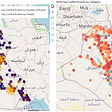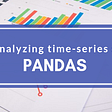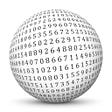# Descriptive Statistics

Descriptive statistics are used to describe the basic features of the data in a study. They provide simple summaries about the sample and the measures. Together with simple graphics analysis, they form the basis of virtually every quantitative analysis of data.

Descriptive Statistics are used to present quantitative descriptions in a manageable form. In a research study, we may have lots of measures. Or we may measure a large number of people on any measure. Descriptive statistics help us to simplify large amounts of data in a sensible way. Each descriptive statistic reduces lots of data into a simpler summary. For instance, consider a simple number used to summarize how well a batter is performing in baseball, the batting average.

# What is Statistics?

Statistics is the science of collecting data and analyzing them to infer proportions (sample) that are representative of the population. In other words, statistics are interpreting data in order to make predictions for the population.

Branches of Statistics:

There are two branches of Statistics.

• DESCRIPTIVE STATISTICS: Descriptive Statistics is a statistic or a measure that describes the data.
• INFERENTIAL STATISTICS: Using a random sample of data taken from a population to describe and make inferences about the population is called Inferential Statistics.

# Commonly Used Measures

1. Measures of Central Tendency
2. Measures of Dispersion (or Variability)

# Measures of Central Tendency

A Measure of Central Tendency is a one-number summary of the data that typically describes the center of the data. This one number summary is of three types.

1. Mean: Mean is defined as the ratio of the sum of all the observations in the data to the total number of observations. This is also known as Average. Thus mean is a number around which the entire data set is spread.
1. Median: Median is the point that divides the entire data into two equal halves. One-half of the data is less than the median, and the other half is greater than the same. Median is calculated by first arranging the data in either ascending or descending order.
• If the number of observations is odd, the median is given by the middle observation in the sorted form.
• If the number of observations is even, the median is given by the mean of the two middle observations in the sorted form.

An important point to note that the order of the data (ascending or descending) does not affect the median.

3. Mode: Mode is the number that has the maximum frequency in the entire data set, or in other words, the mode is the number that appears the maximum number of times. Data can have one or more than one mode.

• If there is only one number that appears a maximum number of times, the data has one mode and is called Uni-modal.
• If there are two numbers that appear maximum number of times, the data has two modes, and is called Bi-modal.
• If there are more than two numbers that appear a maximum number of times, the data has more than two modes and is called Multi-modal.

Example to compute the Measures of Central Tendency

Consider the following data points.

17, 16, 21, 18, 15, 17, 21, 19, 11, 23

• Mean — Mean is calculated as
• Median — To calculate the Median, let's arrange the data in ascending order.

11, 15, 16, 17, 17, 18, 19, 21, 21, 23

Since the number of observations is even (10), the median is given by the average of the two middle observations (5th and 6th here).

• Mode — Mode is given by the number that occurs maximum number of times. Here, 17 and 21 both occur twice. Hence, this is Bimodal data and the modes are 17 and 21.

Note-

1. Since Median and Mode do not take all the data points for calculations, these are robust to outliers, i.e. these are not affected by outliers.
2. At the same time, the Mean shifts towards the outlier as it considers all the data points. This means if the outlier is big, mean overestimates the data and if it is small, the data is underestimated.
3. If the distribution is symmetrical, Mean = Median = Mode. Normal distribution is an example.

# Measures of Dispersion (or Variability)

Measures of Dispersion describes the spread of the data around the central value (or the Measures of Central Tendency)

1. Absolute Deviation from Mean — The Absolute Deviation from Mean, also called Mean Absolute Deviation (MAD), describes the variation in the data set, in the sense that it tells the average absolute distance of each data point in the set. It is calculated as

2. Variance — Variance measures how far are data points spread out from the mean. A high variance indicates that data points are spread widely and a small variance indicates that the data points are closer to the mean of the data set. It is calculated as

3. Standard Deviation — The square root of Variance is called the Standard Deviation. It is calculated a

4. Range — Range is the difference between the maximum value and the minimum value in the data set. It is given as

5. Quartiles — Quartiles are the points in the data set that divides the data set into four equal parts. Q1, Q2, and Q3 are the first, second, and third quartiles of the data set.

• 25% of the data points lie below Q1 and 75% lie above it.
• 50% of the data points lie below Q2 and 50% lie above it. Q2 is nothing but Median.
• 75% of the data points lie below Q3 and 25% lie above it.

6. Skewness — The measure of asymmetry in a probability distribution is defined by Skewness. It can either be positive, negative, or undefined.

• Positive Skew — This is the case when the tail on the right side of the curve is bigger than that on the left side. For these distributions, the mean is greater than the mode.
• Negative Skew — This is the case when the tail on the left side of the curve is bigger than that on the right side. For these distributions, the mean is smaller than the mode.

The most commonly used method of calculating Skewness is

If the skewness is zero, the distribution is symmetrical. If it is negative, the distribution is Negatively Skewed and if it is positive, it is Positively Skewed.

7. Kurtosis — Kurtosis describes whether the data is light-tailed (lack of outliers) or heavy-tailed (outliers present) when compared to a Normal distribution. There are three kinds of Kurtosis:

• Mesokurtic — This is the case when the kurtosis is zero, similar to the normal distributions.
• Leptokurtic — This is when the tail of the distribution is heavy (outlier present) and kurtosis is higher than that of the normal distribution.
• Platykurtic — This is when the tail of the distribution is light( no outlier) and kurtosis is lesser than that of the normal distribution.

--

--

--

## More from Nerd For Tech

NFT is an Educational Media House. Our mission is to bring the invaluable knowledge and experiences of experts from all over the world to the novice. To know more about us, visit https://www.nerdfortech.org/.

## Visualizing DC Real-Time Traffic During The Curfew After Riots## From SPSS to R, Rewriting Code for Survey Data Tabulation: MICS Survey Example## Level Up Your Visualizations: Make Interactive Maps with Python and Bokeh## Post-Negative Oil: The 1-Year Curvature as a measure of Forward Curve Evolution## Analyzing time series data in Pandas## Do You Know Your Critical Data?## How to scrape Google Maps## The Power of a Free-Market Economy## Clandestine Hearts## 210 REASoNS